Question

# Consider the following data for a dependent variable y and two independent variables, x1 and x2....

Consider the following data for a dependent variable y and two independent variables,

x1 and x2.

x1

x2

y
30 12 96
47 10 108
25 17 112
51 16 178
40 5 94
51 19 175
74 7 170
36 12 117
59 13 142
76 16 211

The estimated regression equation for these data is

ŷ = −17.33 + 2.00x1 + 4.73x2.

Here, SST = 15,002.1, SSR = 13,887.5, sb1 = 0.2454, and sb2 = 0.9417.

Find the value of the test statistic. (Round your answer to two decimal places.)

Is β1 significant? Use α = 0.05.

Find the value of the test statistic. (Round your answer to two decimal places.)

Is β2 significant? Use α = 0.05.

Find the value of the test statistic. (Round your answer to two decimal places.)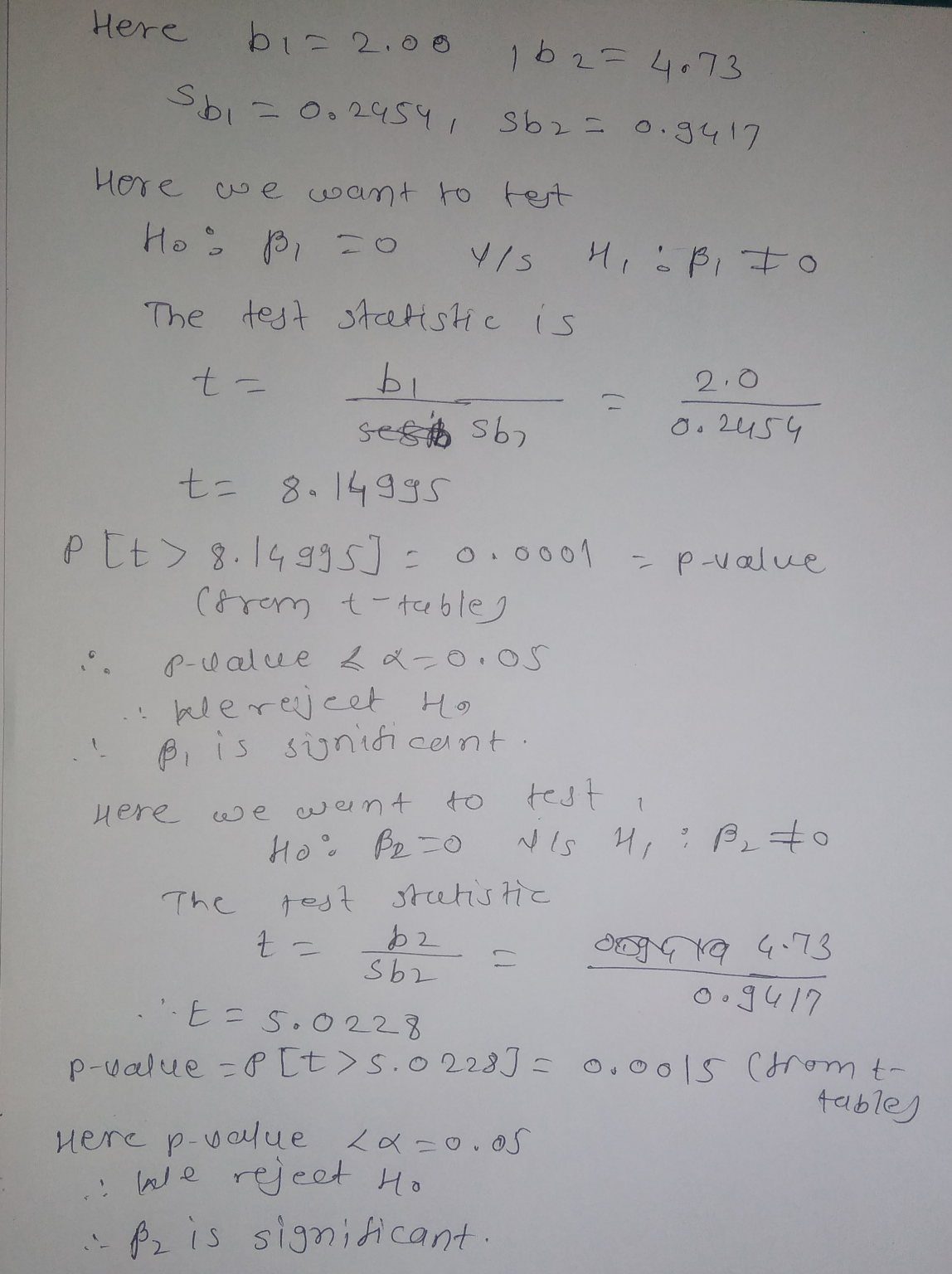Hi dear ,

I have given my 100% to solve your query can you please like it

#### Earn Coins

Coins can be redeemed for fabulous gifts.

Similar Homework Help Questions
• ### Consider the following data for a dependent variable y and two independent variables, x1 and x2....

Consider the following data for a dependent variable y and two independent variables, x1 and x2. x1 x2 y 30 12 94 47 10 108 25 17 112 51 16 178 40 5 94 51 19 175 74 7 170 36 12 117 59 13 142 76 16 211 The estimated regression equation for the data is ŷ = −18.4 + 2.01x1 + 4.74x2. (a) Develop a 95% confidence interval for the mean value of y when x1 = 65...

• ### A statistical program is recommended. Consider the following data for two variables, x and y. x...

A statistical program is recommended. Consider the following data for two variables, x and y. x 22 24 26 30 35 40 y 13 20 33 35 40 36 (a) Develop an estimated regression equation for the data of the form ŷ = b0 + b1x. (Round b0 to one decimal place and b1 to three decimal places.) ŷ = (b) Use the results from part (a) to test for a significant relationship between x and y. Use α =...

• ### You may need to use the appropriate technology to answer this question. In a regression analysis ...

You may need to use the appropriate technology to answer this question. In a regression analysis involving 27 observations, the following estimated regression equation was developed. ŷ = 25.2 + 5.5x1 For this estimated regression equation SST = 1,550 and SSE = 550. (a) At α = 0.05, test whether x1 is significant. State the null and alternative hypotheses. H0: β1 = 0 Ha: β1 ≠ 0H0: β0 = 0 Ha: β0 ≠ 0     H0: β1 ≠ 0 Ha: β1...

• ### Consider the following data for a dependent variable and two independent variables x1 and x2 ....

Consider the following data for a dependent variable and two independent variables x1 and x2 . x1 x2 y 30 13 94 46 11 109 25 17 113 51 16 179 40 5 95 52 19 175 74 7 171 37 13 118 59 14 142 76 17 211 Round your all answers to two decimal places. Enter negative values as negative numbers, if necessary. a. Develop an estimated regression equation relating y to x1 . Predict y if x1=35...

• ### Consider the following data for a dependent variable y and two independent variables, x1 and x2...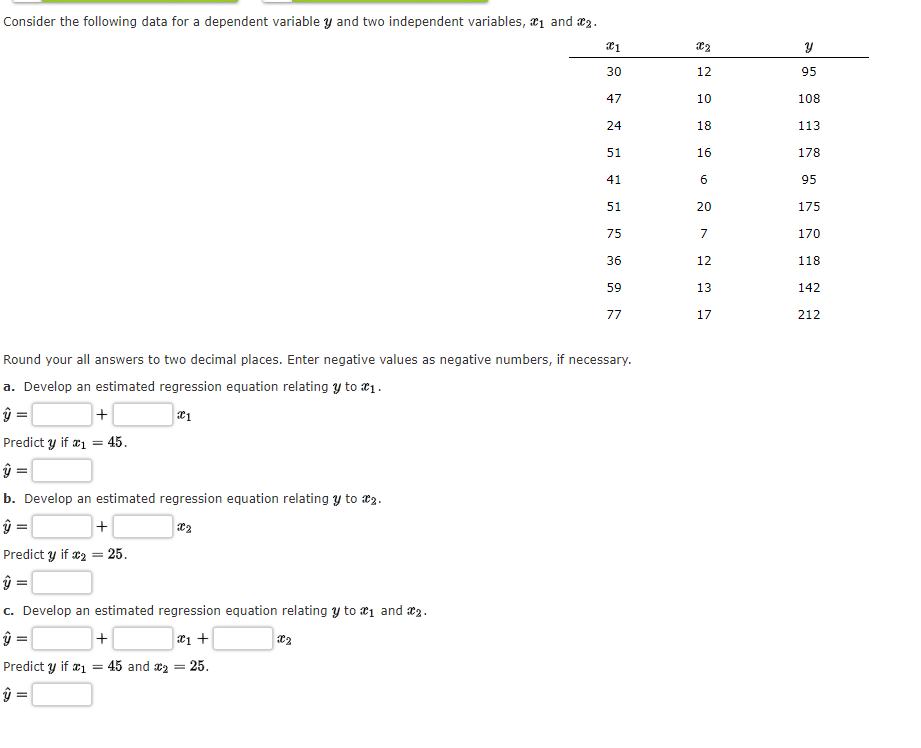Consider the following data for a dependent variable y and two independent variables, x1 and x2 30 12. 95 47 10 108 24 18 113 51 16 178 41 6 95 51 20 175 75 7 170 36 12 118 142 59 13 77 17 212 Round your all answers to two decimal places. Enter negative values as negative numbers, if necessary. a. Develop an estimated regression equation relating y to ai 1 Predict y if 1 = 45 b....

• ### are these right? Consider the following data for a dependent variable y and two independent variables,...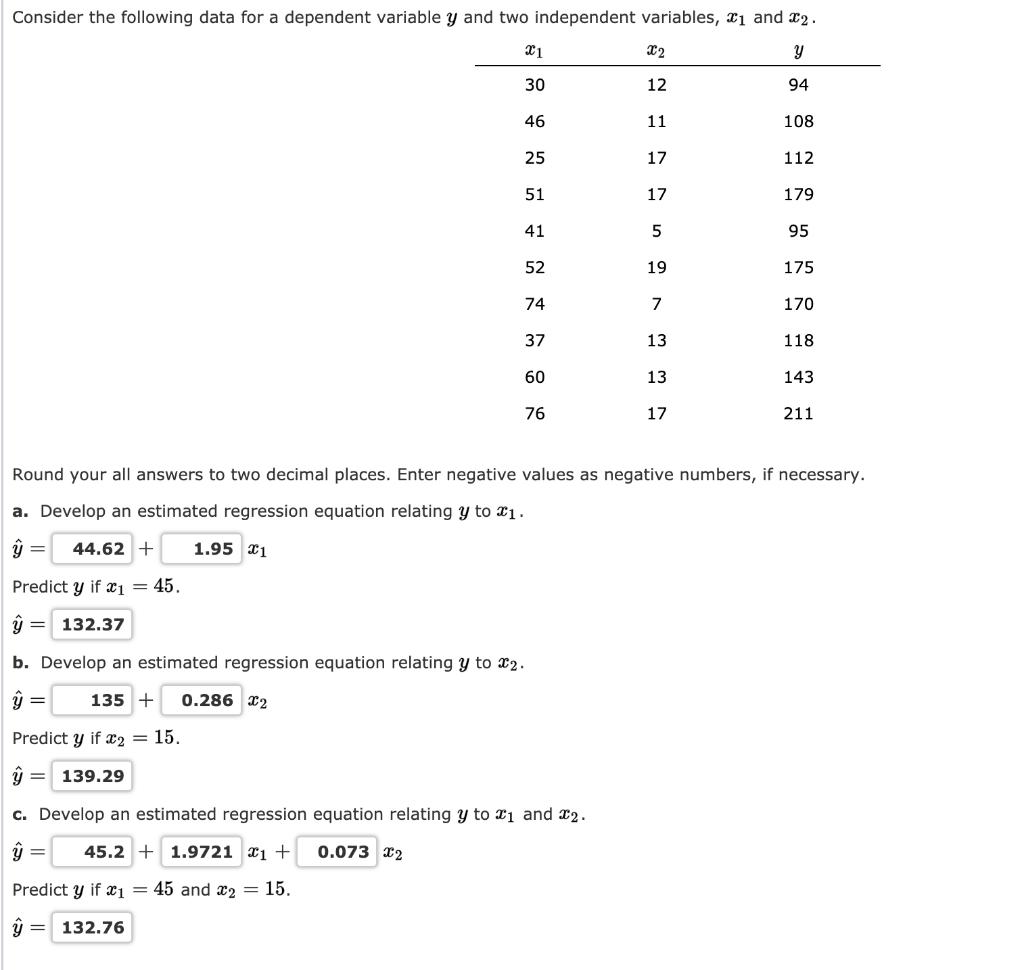are these right? Consider the following data for a dependent variable y and two independent variables, 31 and 22. 94 108 112 179 95 175 170 118 143 211 Round your all answers to two decimal places. Enter negative values as negative numbers, if necessary. a. Develop an estimated regression equation relating y to X1. ŷ = 44.62 + 1.95 21 Predict y if x1 = 45. ŷ = 132.37 b. Develop an estimated regression equation relating y to 22....

• ### Consider the following data for a dependent variable y and two independent variables, 31 and 22....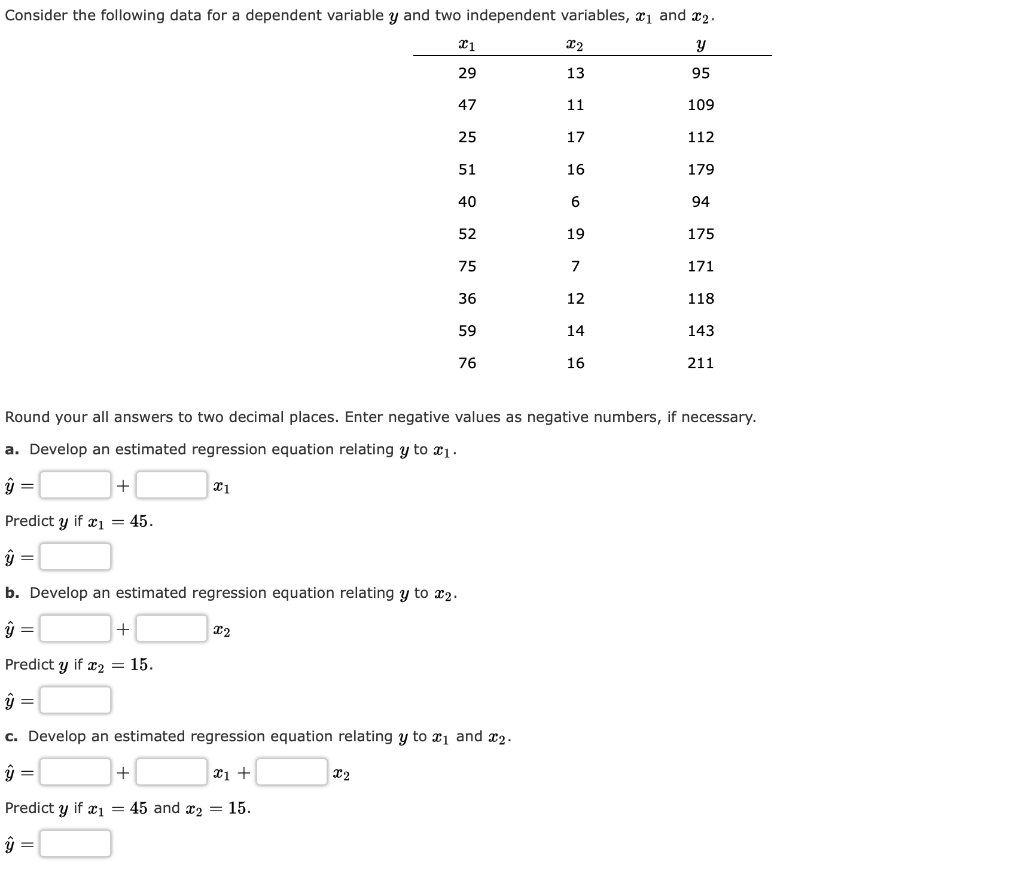Consider the following data for a dependent variable y and two independent variables, 31 and 22. 94 175 171 118 143 211 Round your all answers to two decimal places. Enter negative values as negative numbers, if necessary. a. Develop an estimated regression equation relating y to 21. y = Predict y if x1 = 45. b. Develop an estimated regression equation relating y to 22. ŷ= + 12 Predict y if x2 = 15. = c. Develop an estimated...

• ### Consider the following data for a dependent variable y and two independent variables, 21 and 22....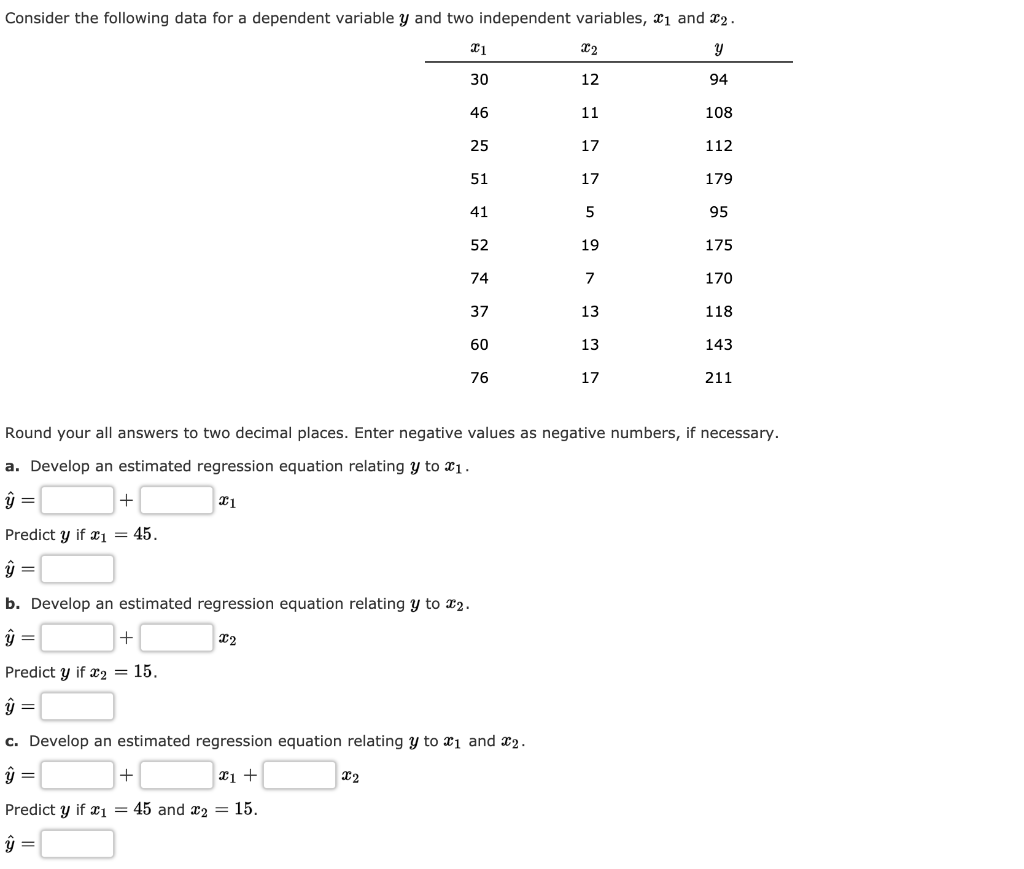Consider the following data for a dependent variable y and two independent variables, 21 and 22. 108 112 179 170 118 143 211 Round your all answers to two decimal places. Enter negative values as negative numbers, if necessary. a. Develop an estimated regression equation relating y to X1. û = 11 Predict y if x1 = 45. ŷ= b. Develop an estimated regression equation relating y to 22. Predict y if X2 = 15. ŷ= c. Develop an estimated...

• ### The following regression output was obtained from a study of architectural firms. The dependent variable is...

The following regression output was obtained from a study of architectural firms. The dependent variable is the total amount of fees in millions of dollars. Predictor Coefficient SE Coefficient t p-value Constant 9.048 3.135 2.886 0.010 x1 0.284 0.111 2.559 0.000 x2 − 1.116 0.581 − 1.921 0.028 x3 − 0.194 0.189 − 1.026 0.114 x4 0.583 0.336 1.735 0.001 x5 − 0.025 0.026 − 0.962 0.112 Analysis of Variance Source DF SS MS F p-value Regression 5 1,895.93 379.2...

• ### Consider the following data for a dependent variable y and two independent variables, 31 and 22....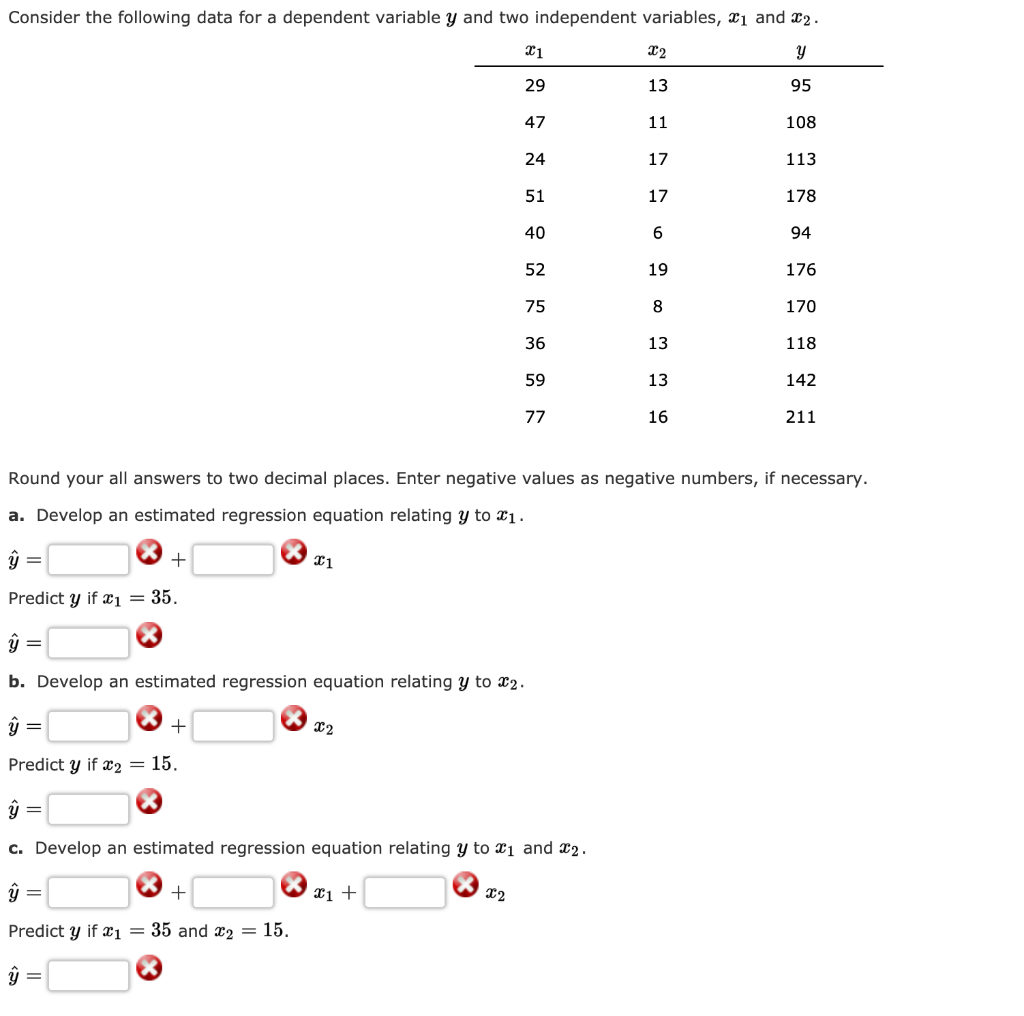Consider the following data for a dependent variable y and two independent variables, 31 and 22. 108 113 178 94 176 170 118 142 211 Round your all answers to two decimal places. Enter negative values as negative numbers, if necessary. a. Develop an estimated regression equation relating y to Xi. Predict y if Xı = 35. y = b. Develop an estimated regression equation relating y to 22. ĝ= + x2 Predict y if x2 = 15. ŷ =...